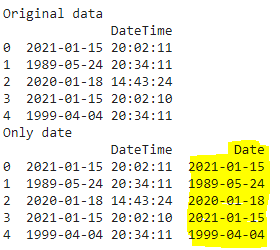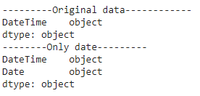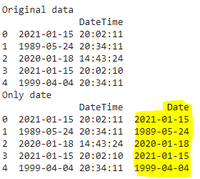# How to Convert Datetime to Date in Pandas ?

• Last Updated : 09 Dec, 2021

DateTime is a collection of dates and times in the format of “yyyy-mm-dd HH:MM:SS” where yyyy-mm-dd is referred to as the date and HH:MM:SS is referred to as Time.

• yyyy stands for year
• mm stands for  month
• dd stands for date
• HH stands for hours
• MM stands for minutes
• SS stands for seconds.

In this article, we are going to discuss converting DateTime to date in pandas. For that, we will extract the only date from DateTime using Pandas Python module.

Syntax:

pd.DataFrame(data)

where data is the input DateTime data.

Example: Python program to create the pandas dataframe with 5 datetime values and display

## Python3

 `# importing pandas module``import` `pandas as pd` `# create pandas DataFrame with one column with five``# datetime values through a dictionary``df ``=` `pd.DataFrame({``'DateTime'``: [``'2021-01-15 20:02:11'``,``                                ``'1989-05-24 20:34:11'``,``                                ``'2020-01-18 14:43:24'``,``                                ``'2021-01-15 20:02:10'``,``                                ``'1999-04-04 20:34:11'``]})` `# display``print``(df)`

Output:

DateTime

0  2021-01-15 20:02:11

1  1989-05-24 20:34:11

2  2020-01-18 14:43:24

3  2021-01-15 20:02:10

4  1999-04-04 20:34:11

## Method 1 : Using date function

By using date method along with pandas we can get date.

Syntax:

dataframe[‘Date’] = pd.to_datetime(dataframe[‘DateTime’]).dt.date

where,

• dataframe is the input dataframe
• to_datetime is the function used to convert datetime string to datetime
• DateTime is the datetime column in the dataframe
• dt.date is used to convert datetime to date
• Date column is the new column to get the date from the datetime

Example: Python program to convert datetime to date using pandas through date function

## Python3

 `# importing pandas module``import` `pandas as pd` `# create pandas DataFrame with one column with five``# datetime values through a dictionary``df ``=` `pd.DataFrame({``'DateTime'``: [``'2021-01-15 20:02:11'``,``                                ``'1989-05-24 20:34:11'``,``                                ``'2020-01-18 14:43:24'``,``                                ``'2021-01-15 20:02:10'``,``                                ``'1999-04-04 20:34:11'``]})` `print``(``"Original data"``)``print``(df)` `# convert datetime column to just date``df[``'Date'``] ``=` `pd.to_datetime(df[``'DateTime'``]).dt.date` `# display``print``(``"Only date"``)``print``(df)`

Output:we can also get the datatypes by using dtypes

Example: Python program to get the datatypes

## Python3

 `# importing pandas module``import` `pandas as pd` `# create pandas DataFrame with one column with five``# datetime values through a dictionary``df ``=` `pd.DataFrame({``'DateTime'``: [``'2021-01-15 20:02:11'``,``                                ``'1989-05-24 20:34:11'``,``                                ``'2020-01-18 14:43:24'``,``                                ``'2021-01-15 20:02:10'``,``                                ``'1999-04-04 20:34:11'``]})` `print``(``"---------Original data------------"``)``print``(df.dtypes)` `# convert datetime column to just date``df[``'Date'``] ``=` `pd.to_datetime(df[``'DateTime'``]).dt.date` `# display``print``(``"--------Only date---------"``)``print``(df.dtypes)`

Output:## Method 2 : using normalize() method

we can get by also using normalize() method, this method is used to normalize the data by extracting the date from DateTime. We are using normalize() method to get the data through pandas

Syntax:

dataframe[‘Date’] = pd.to_datetime(dataframe[‘DateTime’]).dt.normalize()

where,

• dataframe is the input dataframe
• to_datetime is the function used to convert datetime string to datetime
• DateTime is the datetime column in the dataframe
• dt.normalize() is the function which  is used to convert datetime to date
• Date column is the new column to get the date from the datetime

Example: Python code to convert datetime to date using pandas normalize() method.

## Python3

 `# importing pandas module``import` `pandas as pd` `# create pandas DataFrame with one column with five``# datetime values through a dictionary``df ``=` `pd.DataFrame({``'DateTime'``: [``'2021-01-15 20:02:11'``,``                                ``'1989-05-24 20:34:11'``,``                                ``'2020-01-18 14:43:24'``,``                                ``'2021-01-15 20:02:10'``,``                                ``'1999-04-04 20:34:11'``]})` `print``(``"Original data"``)``print``(df)` `# convert datetime column to just date using normalize()``# method``df[``'Date'``] ``=` `pd.to_datetime(df[``'DateTime'``]).dt.normalize()` `# display``print``(``"date extracted"``)``print``(df)`

Output:My Personal Notes arrow_drop_up